# 修飾符

`g` 全域性

`i` 不區分大小寫

`m` 多行模式

`s` 啟用 “dotall” 模式，允許點 `.` 匹配換行符 `\n`

`u` 開啟完整的 Unicode 支援，該修飾符能夠正確處理代理對

`y` 粘滯模式，在文字中的確切位置搜尋 ```js let str = "we we W WE we dWEd dWE dwed wed ddwe" console.log( str.match(/we/gi) ) // 表示全域性搜尋 we 不區分大小寫 // /we/i/ 表示匹配第一個 we, 沒有匹配項，不會收到一個空陣列，而是會收到 null // 如果希望保底為陣列 console.log( str.match(/we/i ) || [] )

// 替換 console.log( "we we W WE we dWEd dWE dwed wed ddwe".replace(/we/i, "hone") )

// 找到至少一個匹配項則返回 true，否則返回 false /we/i.test(str) // true ```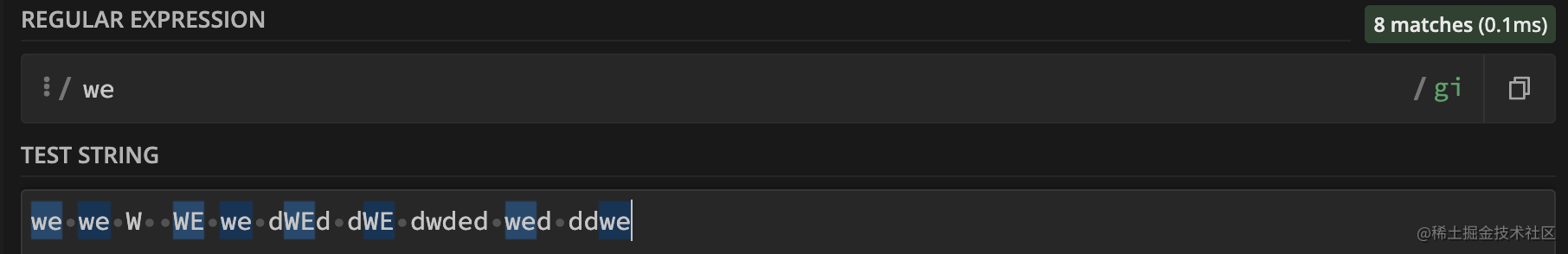# 字元類

| 正則 | 含義 | | --- | --- | | `/\d/` | 數字：0-9 | |`/\s/` | 空格符號：包括空格，製表符 `\t`，換行符 `\n` 和其他少數稀有字元，例如 `\v``\f` 和 `\r` | |`/\w/` | 「單字」字元：拉丁字母或數字或下劃線 `_`。非拉丁字母（如西裡爾字母或印地文）不屬於 `\w`|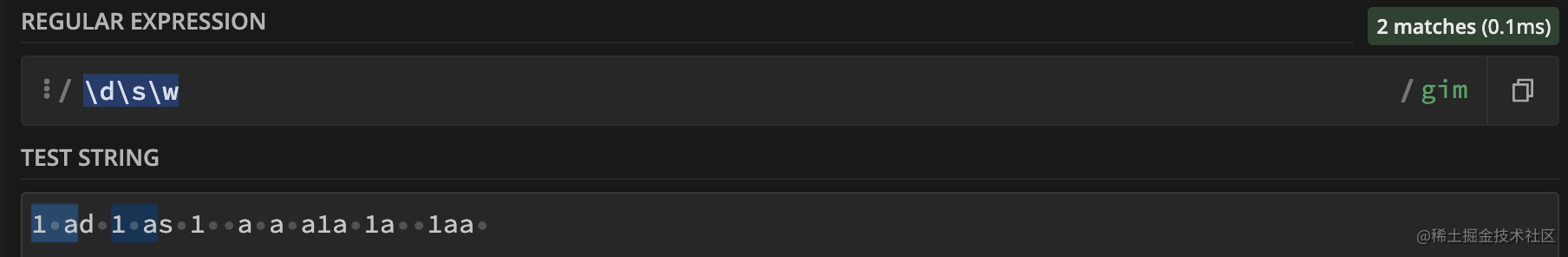```let str = "+7(903)-123-45-67" console.log( str.match(/\d/) ) // 7 匹配第一個 console.log( str.match(/\d/g) ).join('') // 查詢所有,最終結果 79031234567 str.replace(/\D/g, "") // 查詢非數字並刪除,最終結果 79031234567```

## 反向類

| 正則 | 含義 | | --- | --- | | `/\D/`| 除 `/d` 以外的任何字元 | |`/\S/` | 除 `/s` 以外的任何字元 | |`/\W/` | 除 `/w` 以外的任何字元 |

## 點（.）匹配「任何字元」

`.` 它與除換行符之外的任何字元匹配, 預設情況下，點與換行符 `\n` 不匹配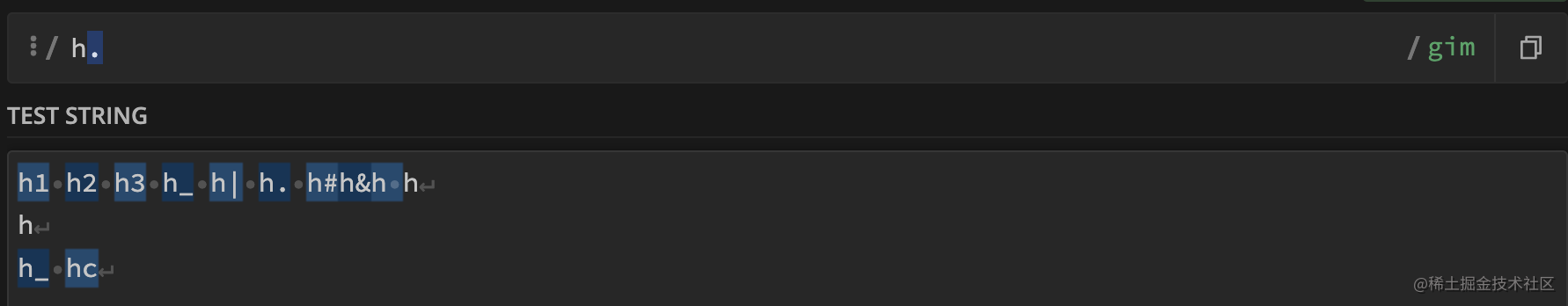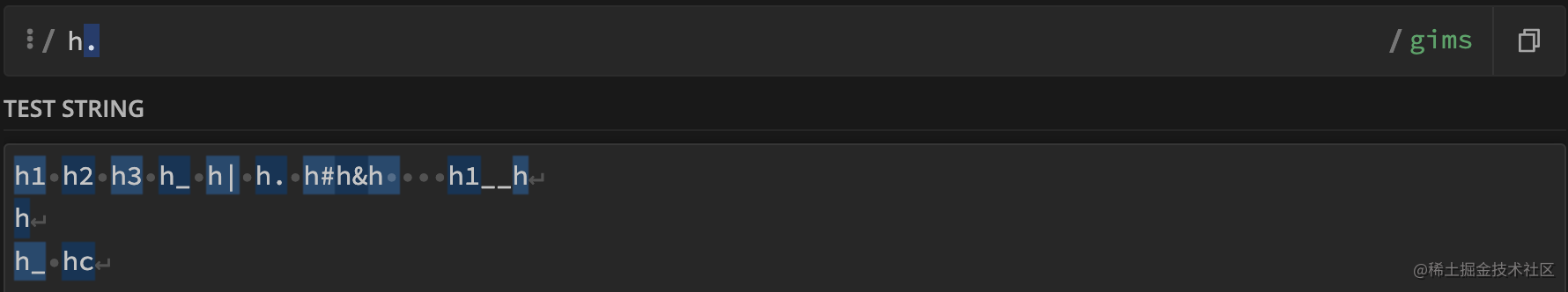# Unicode：修飾符 "u" 和類 \p{...}

## Unicode 屬性 \p{…}

1. 4 個位元組長的字元被以正確的方式處理：被看成單個字元，而不是 2 個 2 位元組長的字元。
2. Unicode 屬性可以被用於查詢：`\p{…}`

# 錨點：字串開始 ^ 和末尾 \$

## 測試完全匹配

```js // 所對應的匹配項必須正好在文字 '^' 的開頭之後開始，並且結尾 '\$' 必須緊跟其後 "12:24".test(/^\d\d:\d\d\$/) // 可以匹配到``` 錨點 `^` 和 `\$` 屬於測試,它們的寬度為零。

# 錨點 ^ \$ 的多行模式，修飾符 "m"

`m` 啟用多行模式修飾符 ，可以匹配每一行的`^``\$`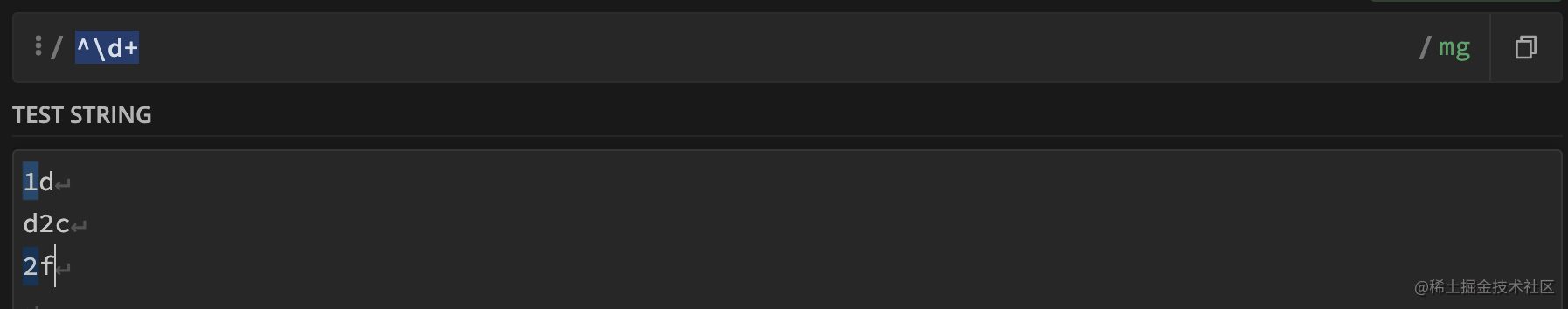預設情況下，錨點 `^` 僅匹配文字的開頭，在多行模式下，它匹配行的開頭

## 搜尋行的末尾 \$

`\d\$` 尋找每行的最後一個數字。

## 尋找新的一行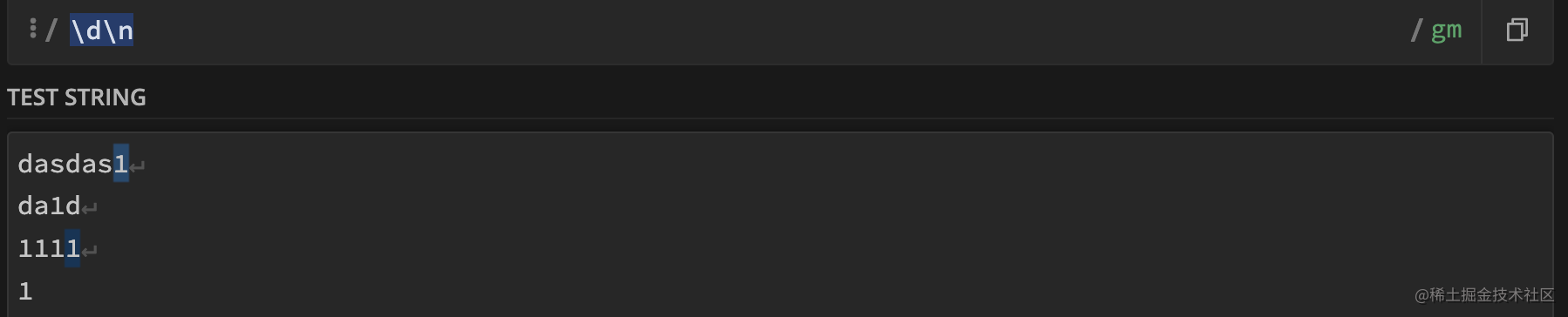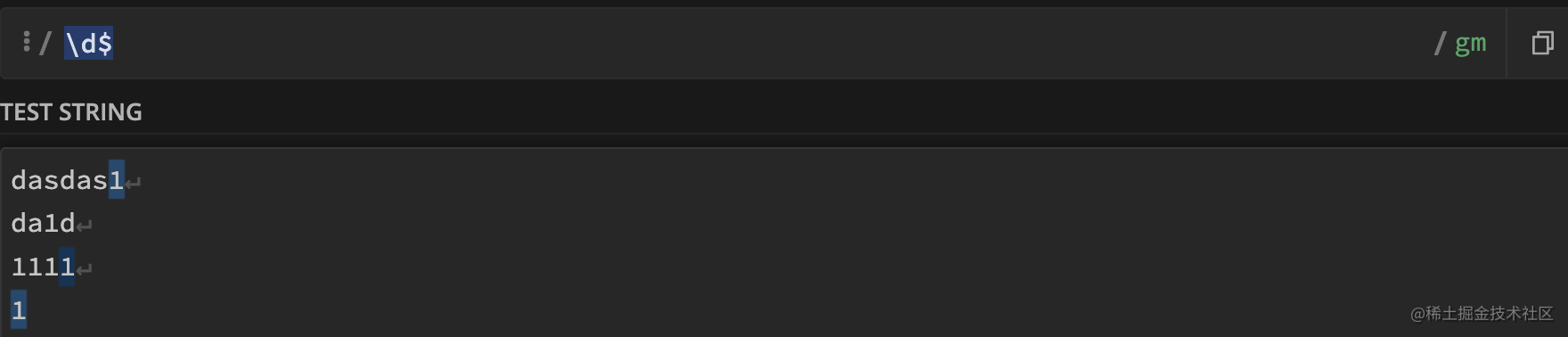# 詞邊界：\b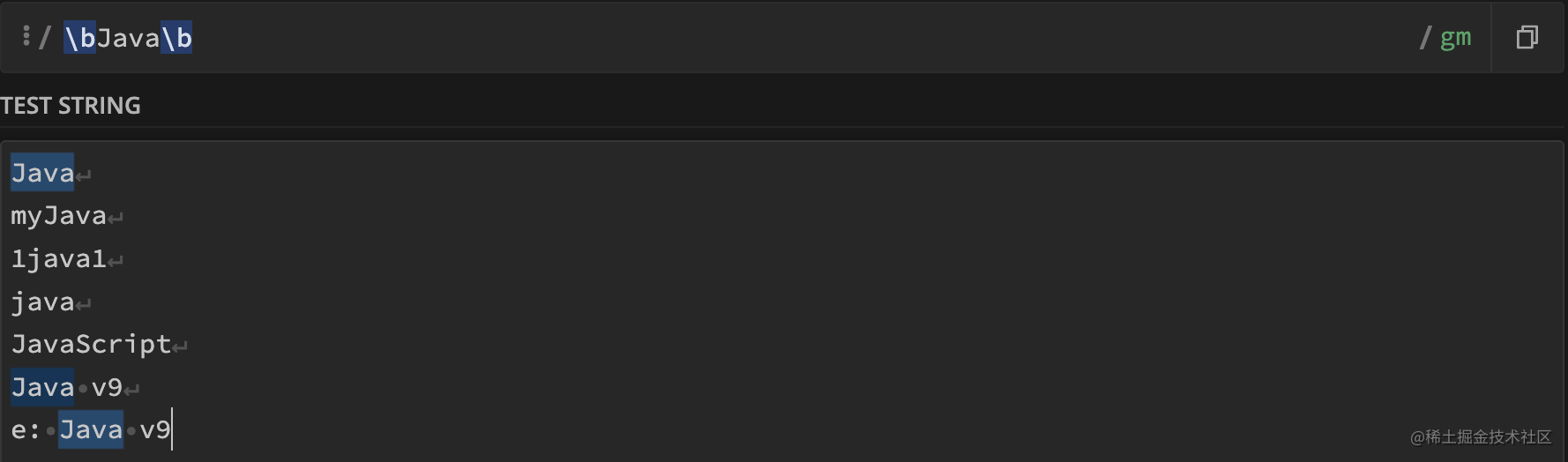⚠️注意： 詞邊界 `\b` 不適用於非拉丁字母

# 轉義，特殊字元

## 轉義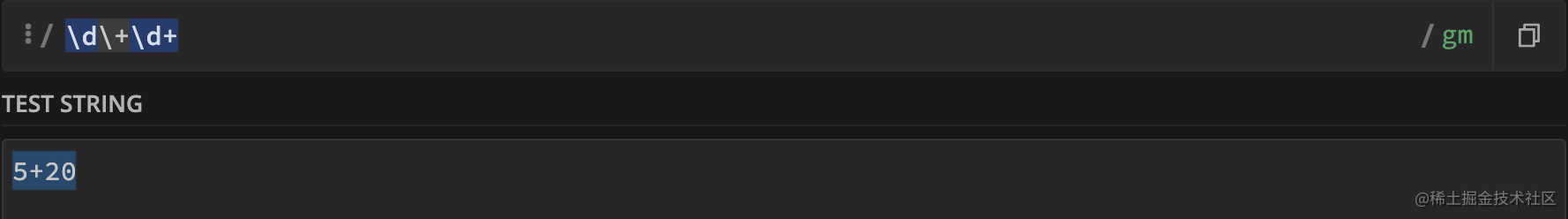- 要在字面意義上搜索特殊字元 `[ \ ^ \$ . | ? * + ( )`，我們需要在它們前面加上一個反斜槓 `\`（“轉義它們”）。

# 集合和範圍 [...]

## 集合

`[…]`  表示查詢滿足的 "任意一個" 。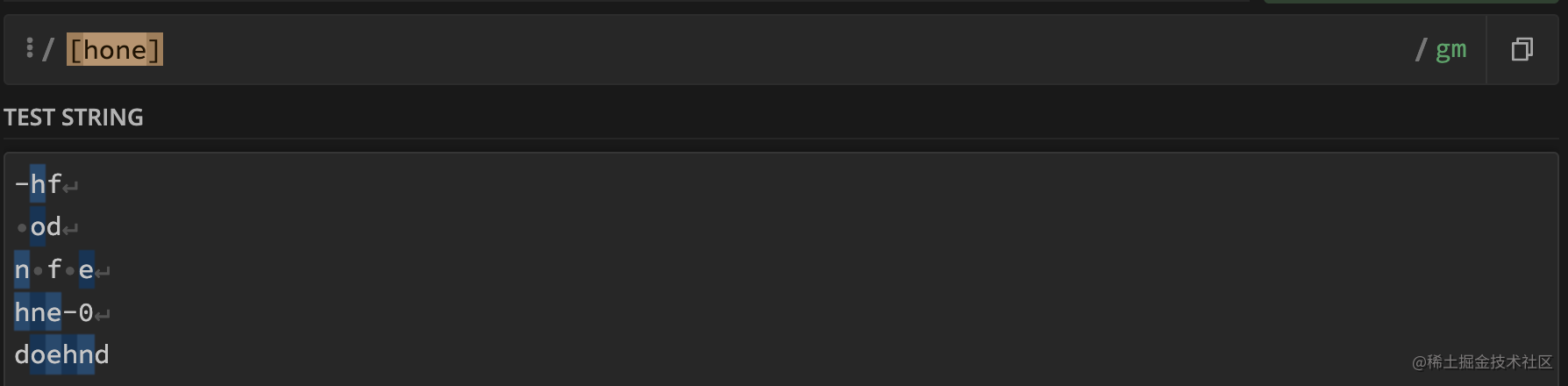## 範圍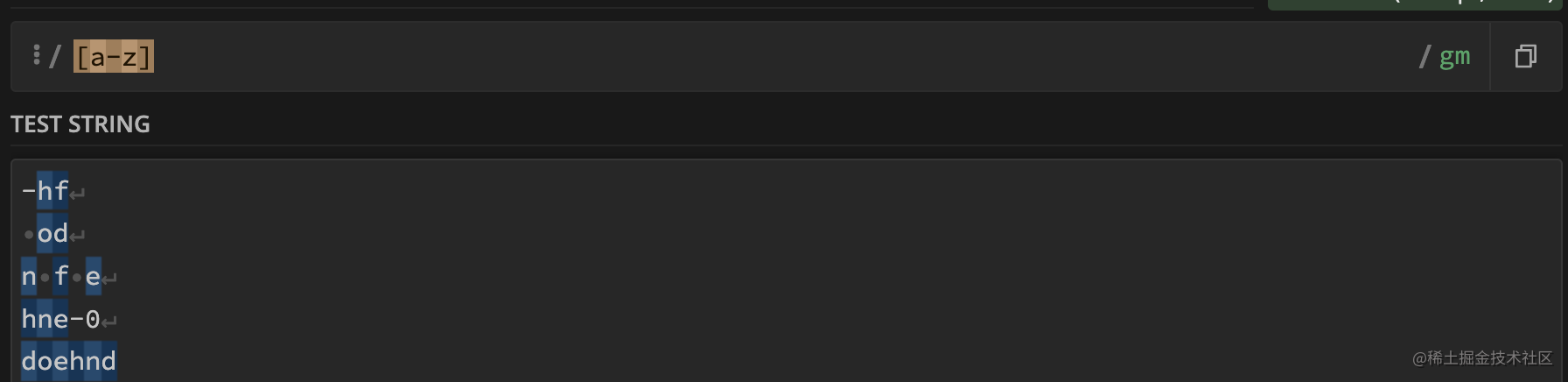## 排除範圍

`[^…]` "排除"範圍匹配

• `[^aeyo]` —— 匹配除了 `'a'``'e'``'y'` 或 `'o'` 之外的任何字元。
• `[^0-9]` —— 匹配除了數字之外的任何字元，與 `\D` 作用相同。
• `[^\s]` —— 匹配任何非空格字元，與 `\S` 作用相同
• `[^]` —— 表示任意字元，即對空值取反，得到全集

## 範圍和修飾符 “u”

// 忘記寫 u '𝒳'.match(/[𝒳-𝒴]/); // Error: Invalid regular expression ```

# 量詞 +, *, ? 和 {n}

## {n}

`\d{5}` 表示 5 位數，與 `\d\d\d\d\d` 相同。

### 確切的位數：`{5}`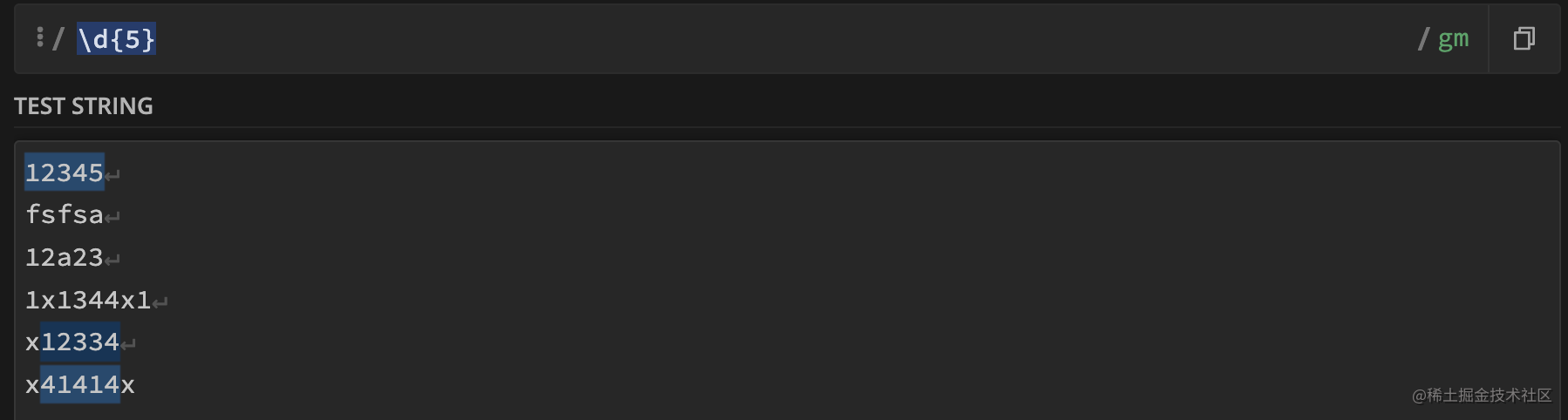### 範圍：`{2,5}`，匹配 2-5 個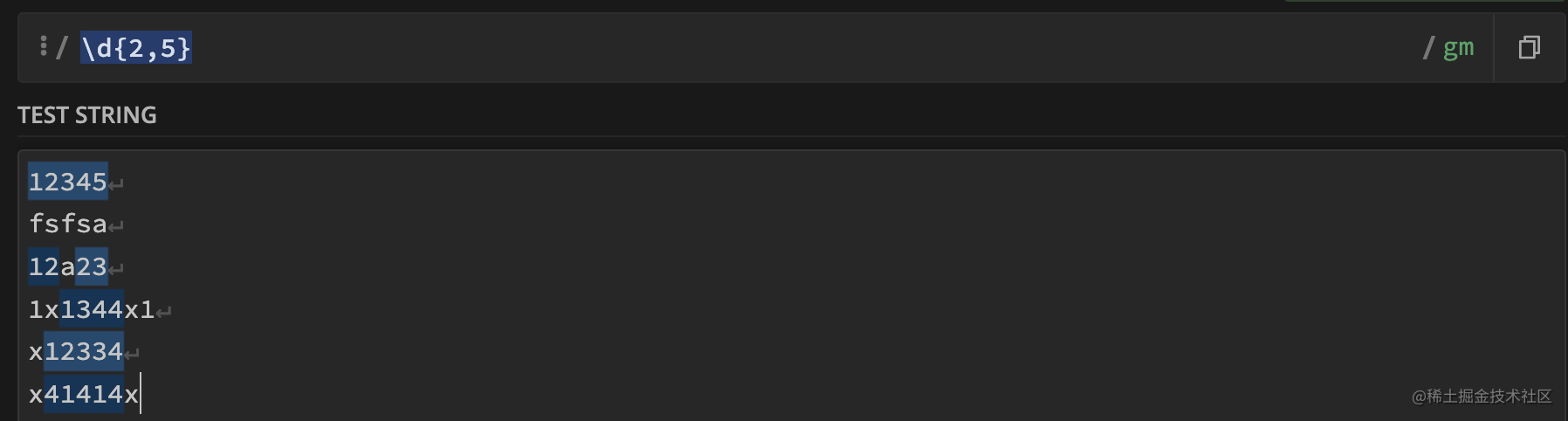`\d{2,}` 查詢大於2的數字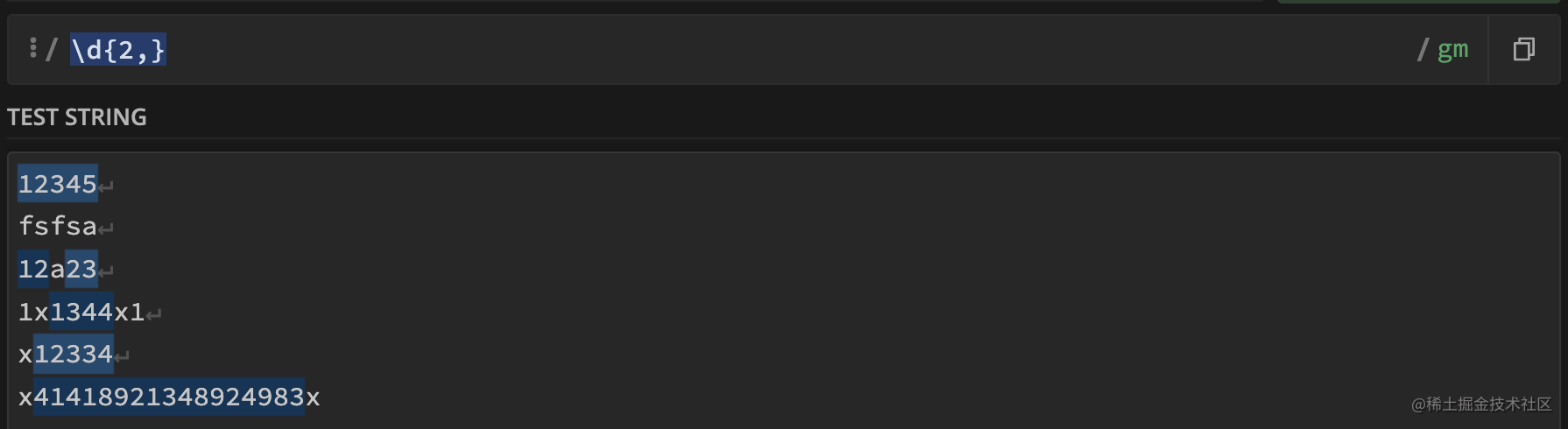# 貪婪量詞和惰性量詞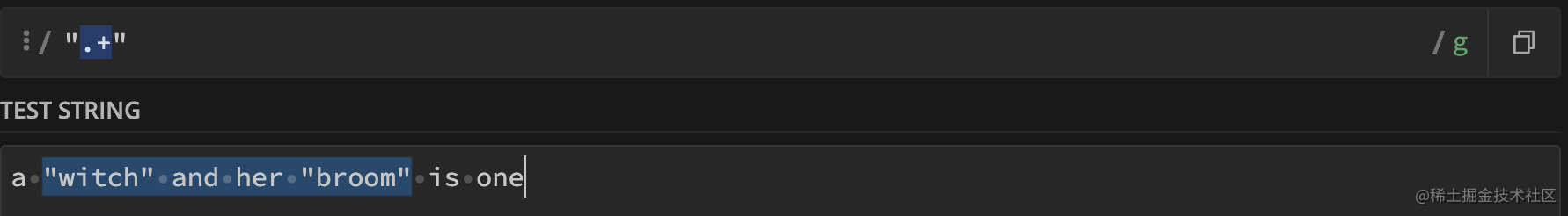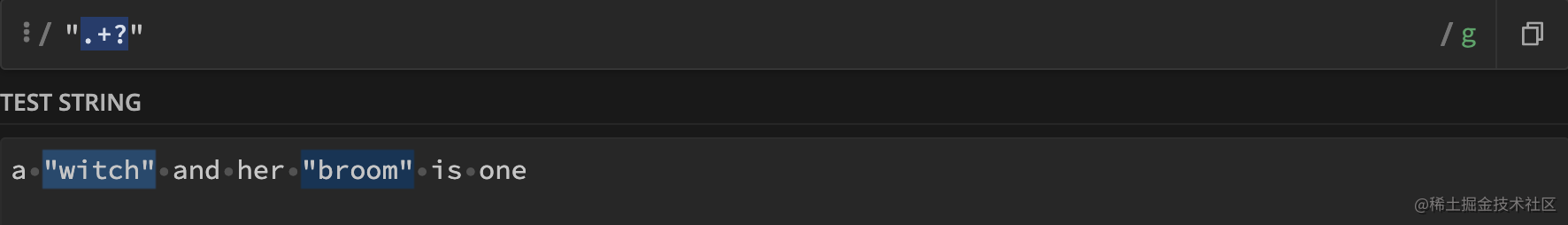# 捕獲組 (...)

• 方法 `str.match` 僅當不帶修飾符 `g` 時返回捕獲組。
• 方法 `str.matchAll` 始終返回捕獲組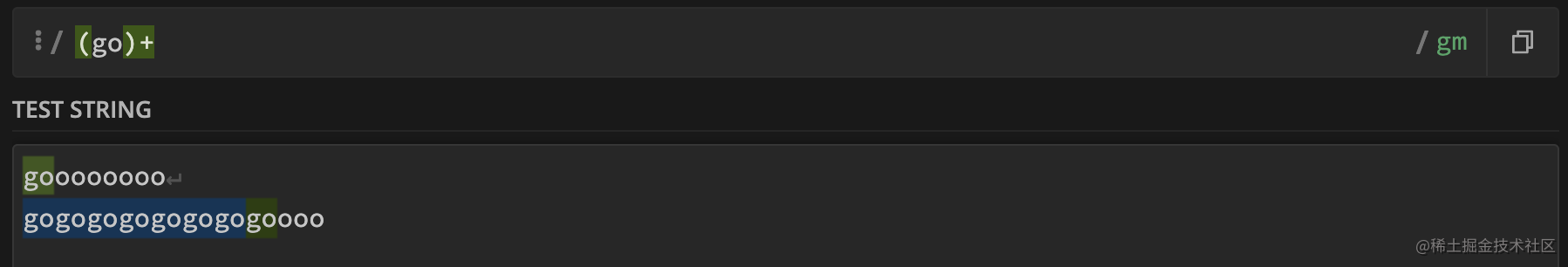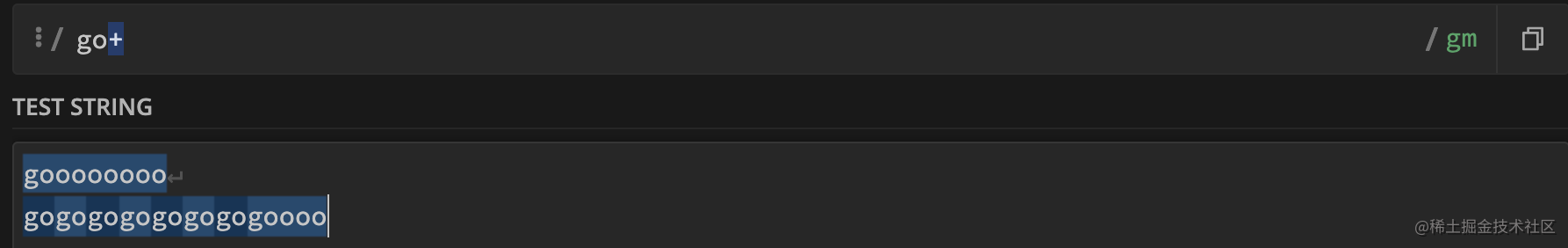```js // 域名搜尋 "site.com my.site.com".match(/(\w+.)+\w+/g) // site.com my.site.com

// 電子郵件 "[email protected] @ [email protected]".match(/[-.\w]+@([\w-]+.)+[\w-]+/g) // [email protected], [email protected] ```

## 巢狀組

```js let str = '<span class="my">' let regexp = /<(([a-z]+)\s*([^>]*))>/ let result = str.match(regexp) alert(result) // <span class="my"> alert(result) // span class="my" alert(result) // span alert(result) // class="my"```

## 可選組

```js 'a'.match(/a(z)?(c)?/)```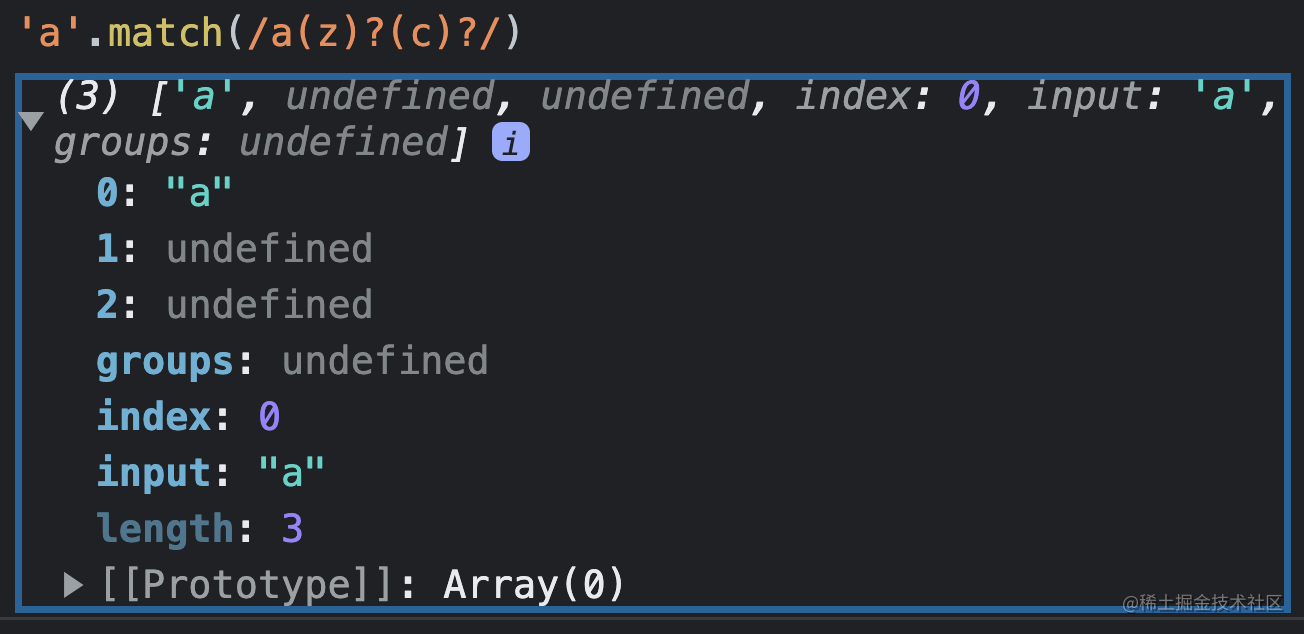## 命名組

```js // 這裡的 就是對每個陣列進行命名 let dateRegexp = /(?[0-9]{4})-(?[0-9]{2})-(?[0-9]{2})/; let str = "2019-04-30";

// 匹配的組在 .groups 屬性中 let groups = str.match(dateRegexp).groups;

## 替換中的捕獲組

`\$n` 中的 `n` 是組號 ```js let str = "John Bull"; let regexp = /(\w+) (\w+)/; // 這裡分成兩組

// \$1 表示 John，\$2 表示 Bull alert( str.replace(regexp, '\$2, \$1') ); // Bull, John

// 命名的括號 引用為 \$ let regexp = /(?[0-9]{4})-(?[0-9]{2})-(?[0-9]{2})/g; let str = "2019-10-30, 2020-01-01";

alert( str.replace(regexp, '\$.\$.\$') ); // 30.10.2019, 01.01.2020 ```

## 非捕獲組

// ?: 從捕獲組中排除 'go' let regexp = /(?:go)+ (\w+)/i; let result = str.match(regexp); alert( result ); // Gogogo John（完整的匹配項） alert( result ); // John alert( result.length ); // 2（在陣列中沒有其他陣列項） ```

# 模式中的反向引用：`\N` 和 `\k<name>`

## 按編號反向引用：`\N`

`````js let str =```He said: "She's the one!".`;

let regexp = /(['"])(.*?)\1/g;

alert( str.match(regexp) ); // "She's the one!" ````` 程式碼執行過程：正則表示式引擎會找到第一個引號```(['"])` 並記住其內容。那是第一個捕獲組。

• 注意：如果我們在捕獲組中使用 `?:`，那麼我們將無法引用它。用 `(?:...)` 捕獲的組被排除，引擎不會記住它。
• 注意：在替換字串中我們使用美元符號：`\$1`，而在模式中 —— 使用反斜槓 `\1`

## 按命名反向引用：`\k<name>`

alert( str.match(regexp) ); // "She's the one!" ```

# 選擇 (OR)

• `gr(a|e)y` 等同於 `gr[ae]y`
• `gra|ey` 表示 `gra` 或 `ey`

# 前瞻斷言與後瞻斷言

## 前瞻斷言

`x(?=y)` 僅在後面是 `Y` 時匹配 `X`

```js "1 turkey costs 30€".match(/\d+(?=€)/) // 30```

## 否定的前瞻斷言

`X(?!Y)`，意思是“搜尋 `X`，但前提是後面沒有 `Y` ```js "1 turkey costs 30€".match(/\d+(?!€)/) // 1```

## 後瞻斷言

• 肯定的後瞻斷言：`(?<=Y)X`，匹配 `X`，僅在前面是 `Y` 的情況下。
• 否定的後瞻斷言：`(?<!Y)X`，匹配 `X`，僅在前面不是 `Y` 的情況下。 ```js "1 turkey costs \$30".match(/(?<=\\$)\d+/) // 30 "1 turkey costs \$30".match(/(?<!\\$)\b\d+/g)) // 1```

## 捕獲組

```js "1 turkey costs 30€".match(/\d+(?=(€))/) // 30, € "1 turkey costs \$30".match(/(?<=(\\$))\d+/) // 30, \$```

# 粘性修飾符 "y"，在位置處搜尋

`y` 修飾符讓我們能夠在源字串中的指定位置進行搜尋 ```js let str = 'let varName = "value"'; let regexp = /\w+/y; regexp.lastIndex = 3; alert( regexp.exec(str) ); // null（位置 3 有一個空格，不是單詞） regexp.lastIndex = 4; alert( regexp.exec(str) ); // varName（在位置 4 的單詞）```

# 正則表示式和字串的方法

## str.match(regexp)

1. 如果 `regexp` 不帶有修飾符 `g`，則它以陣列的形式返回第一個匹配項，其中包含捕獲組和屬性 `index`（匹配項的位置）、`input`（輸入字串，等於 `str`
2. 如果 `regexp` 帶有修飾符 `g`，則它將返回一個包含所有匹配項的陣列，但不包含捕獲組和其它詳細資訊。
3. 如果沒有匹配項，則無論是否帶有修飾符 `g`，都將返回 `null`

## str.matchAll(regexp)

1. 它返回一個包含匹配項的可迭代物件，而不是陣列。我們可以用 `Array.from` 將其轉換為一個常規陣列。
2. 每個匹配項均以一個包含捕獲組的陣列形式返回（返回格式與不帶修飾符 `g` 的 `str.match` 相同）。
3. 如果沒有結果，則返回的是一個空的可迭代物件而不是 `null`

## str.split(regexp|substr, limit)

`alert('12, 34, 56'.split(/,\s*/)) // 陣列 ['12', '34', '56']`

## str.replace(str|regexp, str|func)

```js // 用冒號替換連字元 alert('12-34-56'.replace("-", ":")) // 12:34-56```

## str.replaceAll(str|regexp, str|func)

1. 如果第一個引數是一個字串，它會替換 所有出現的 和第一個引數相同的字串​，​而 `replace` 只會替換 第一個
2. 如果第一個引數是一個沒有修飾符 `g` 的正則表示式，則會報錯。帶有修飾符 `g`，它的工作方式與 `replace` 相同。

`replaceAll` 的主要用途是替換所有出現的字串。

## regexp.exec(str)

`regexp.exec(str)` 方法返回字串 `str` 中的 `regexp` 匹配項。與以前的方法不同，它是在正則表示式而不是在字串上呼叫的。

• 呼叫 `regexp.exec(str)` 會返回第一個匹配項，並將緊隨其後的位置儲存在屬性 `regexp.lastIndex` 中。
• 下一次這樣的呼叫會從位置 `regexp.lastIndex` 開始搜尋，返回下一個匹配項，並將其後的位置儲存在 `regexp.lastIndex` 中。
• ……以此類推。
• 如果沒有匹配項，則 `regexp.exec` 返回 `null`，並將 `regexp.lastIndex` 重置為 `0`